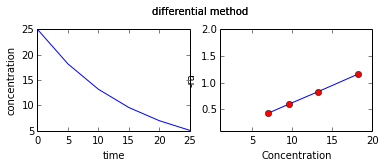# Chapter 7 : Chemical Kinetics¶

### example 7.3 page number 305¶

In :
import math

r = 3**2*3;   #according to the rate reaction
print "reaction reate will be increased by with 3 times increase in pressure = %f times"%(r)

r = 3**2*3;   #according to the rate reaction
print "reaction reate will be increased by with 3 times decrease in volume = %f times"%(r)

r = 3**2;   #according to the rate reaction
print "reaction reate will be increased by with 3 times increase in conc of NO = %f times"%(r)

reaction reate will be increased by with 3 times increase in pressure = 27.000000 times
reaction reate will be increased by with 3 times decrease in volume = 27.000000 times
reaction reate will be increased by with 3 times increase in conc of NO = 9.000000 times


### example 7.10 page number 316¶

In :
from scipy.optimize import fsolve
import math
moles_A = 3.;
moles_B = 5.;
K = 1.;

def F(x):
return 15.-8*x;

x = 10.;
y = fsolve(F,x)

print "amount of A transformed = %f percent"%(y*100/3)

amount of A transformed = 62.500000 percent


### example 7.11 page number 316¶

In :
from scipy.optimize import fsolve
import math
Cp = 0.02;
Cq = 0.02;
K = 4*10**-2;
Cb = 0.05;
Cb_i = Cb+Cp;
a = (Cp*Cq)/(K*Cb);

def F(x):
return x-0.02-a;

x = 10.;
y = fsolve(F,x)

print "conc of A= %f mol/l"%(y)
print "conc of B= %f mol/l"%(Cb_i)

conc of A= 0.220000 mol/l
conc of B= 0.070000 mol/l


### example 7.12 page number 316¶

In :
import math

Ce_N2 = 3.;   #equilibrium conc of N2
Ce_H2 = 9.;   #equilibrium conc of H2
Ce_NH3 = 4.;  #equilibrium conc oh NH3

C_N2 = Ce_N2 + 0.5*Ce_NH3;
C_H2 = Ce_H2 + 1.5*Ce_NH3;

print "concentration of N2 = %f mol/l \nconcentration of H2 = %f mol/l"%(C_N2,C_H2)

n_H2 = 3.;   #stotiometric coefficient
n_N2 = 1.;   #stotiometric coefficient
n_NH3= 2.;   #stotiometric coefficient
delta_n = n_H2+n_N2-n_NH3;
if delta_n > 0:
print  "delta_n =%f  since delta_n is greater than 0,equilibrium will shift to right with increase in volume"%(delta_n)

concentration of N2 = 5.000000 mol/l
concentration of H2 = 15.000000 mol/l
delta_n =2.000000  since delta_n is greater than 0,equilibrium will shift to right with increase in volume


### example 7.13 page number 317¶

In :
from scipy.optimize import fsolve
import math
moles_A = 0.02;
K = 1.;

moles_B = 0.02;
def F(x):
return  moles_A*moles_B-(moles_A+moles_B)*x;

x = 10.;
y = fsolve(F,x)
print "amount of A transformed = %f percent"%(y*100/0.02)

moles_B = 0.1;
y = fsolve(F,x)
print "amount of A transformed = %f percent"%(y*100/0.02)

moles_B = 0.2;
y = fsolve(F,x)
print "amount of A transformed = %.0f percent"%(y*100/0.02)

amount of A transformed = 50.000000 percent
amount of A transformed = 83.333333 percent
amount of A transformed = 91 percent


### example 7.15 page no : 319¶

In :
import math
from numpy import *
from matplotlib.pyplot import *

%matplotlib inline
t = array([0,5,10,15,20,25])
C_A = array([25,18.2,13.2,9.6,7,5.1])

s = 0;
k = zeros(6)

for i in range(1,6):
k[i] = (1./t[i])*math.log(25./C_A[i])
#print  (k[i],"k values for various conc.")
s = s+k[i]

print "average value of k = %f"%(s/5)
print  ("ra =- 0.06367*CA","since its a first order reaction,")

subplot(221)
plot(t,C_A)

xlabel("time")
ylabel("concentration")
suptitle("integral method")

ra = array([1.16,0.83,0.60,0.43])
C_A = array([18.2,13.2,9.6,7])

subplot(222)
plot(C_A,ra)
plot(C_A,ra,'ro')
xlabel("Concentration")
ylabel("-ra")
suptitle("differential method")
xlim(1,20)
ylim(.1,2)
print "rate from differential method = -0.064*CA"

Populating the interactive namespace from numpy and matplotlib
average value of k = 0.063680
('ra =- 0.06367*CA', 'since its a first order reaction,')
rate from differential method = -0.064*CA

WARNING: pylab import has clobbered these variables: ['draw_if_interactive', 'new_figure_manager']
%pylab --no-import-all prevents importing * from pylab and numpy### example 7.16 page no : 322¶

In :
import math
E = 75200.    #in J/mol
E1 = 50100.   #in J/mol
R = 8.314    #in J/mol K
T = 298.   #in K

ratio = math.exp((E1-E)/(R*T));
rate_increase = ratio**-1

print  "increase in rate of reaction =",rate_increase,"times"

increase in rate of reaction = 25106.6042072 times

In [ ]: